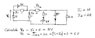# Circuit Analysis: Understand V2 & 3amp Equation

• Engineering
• fran1942

#### fran1942

Hello, I have tried really hard to understand this circuit but I just can't grasp it.

I am trying to understand how V2 comes to 6 volts ie. where does the 3amp come from that is multiplied by the value of V2 to give 6 volts.
It would help a lot if I could see the linear equation which gives 3 amps for the relevant loop.

Thanks kindly for any help with this.

#### Attachments

•layer.jpg
19.3 KB · Views: 323

hello fran1942!use KCL to find the current through the bottom wire, directly under the 2Ω …

how much is that, and how does it help you to find the current through the 2Ω itself?OK thanks. I see that current will be the combination of the 2 and the 1, making 3. Is it as simple as that ?

The main thing bugging me was that I could not work out the linear equation to give that 3amps.
what is worrying you about that?